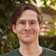# Leetcode - Episode 2 - Three More Easys

January 02, 2019

After warming up (literally, I cycled home in frosty weather) I tackled some more Leetcode problems today.

### 961. N-Repeated Element in Size 2N Array

Problem: Given an list `A` of size `2N`, there are `N+1` unique elements — which one is repeated `N` times?

For this solution I used the high performance Counter that I discovered in my previous blog post.

``````from collections import Counter

class Solution:
def repeatedNTimes(self, A):
"""
:type A: List[int]
:rtype: int
"""
counter = set()
half = len(A) / 2

for i in A:
counter[i] += 1
if counter[i] == half:

return i``````

This solution has a runtime complexity of `O(n)` and a space complexity of `O(n)`. I’m not sure whether this can be improved upon. All elements of the list need to be checked because it is unsorted — we can’t know where our `N` element will be.

Leetcode’s tests for this problem only have unique elements and so a Set is technically faster but I prefer my solution as it’s more ‘correct’ per the problem statement.

### 965. Univalued Binary Tree

Problem: Are all the values of this binary tree the same?

It’s a tree-traversal problem so it’s either going to be Depth-First Search or Breadth-First Search. I chose DFS.

``````# Definition for a binary tree node.
# class TreeNode:
#     def __init__(self, x):
#         self.val = x
#         self.left = None
#         self.right = None

class Solution:
def isUnivalTree(self, root):
"""
:type root: TreeNode
:rtype: bool
"""
value = root.val

def recursive_search(node):
if node == None:
return True
elif node.val != value:
return False
else:
return recursive_search(node.left) and recursive_search(node.right)
return True

return recursive_search(root)``````

Whatever the solution to this problem, the runtime complexity will be `O(n)` as every node must be touched to satisfy the problem condition. We used DFS so the stack will only hold one singular winding tree-root in memory at once. Hence the space complexity is `O(d)` where `d` is the depth of the tree.

### 804. Unique Morse Code Words

Problem: Given a list of lowercase words, how many unique morse code sequences are there?

They’re looking for unique occurrences so a standard optimization is likely to be a Set.

``````class Solution:
def uniqueMorseRepresentations(self, words):
"""
:type words: List[str]
:rtype: int
"""
morse = [".-","-...","-.-.","-..",".","..-.","--.","....","..",".---","-.-",".-..","--","-.","---",".--.","--.-",".-.","...","-","..-","...-",".--","-..-","-.--","--.."]

unique_words = set()

for word in words:
as_morse = []
for c in word:
as_morse.append(morse[ord(c) - 97])

return len(unique_words)``````

My solution has a runtime complexity of `O(n)` with a space complexity of `O(n)`. Similar to Leetcode problem 709, it may be faster to use a Dictionary that maps letters to morse as opposed to performing a calculation for each letter but this may depend on language implementation.

After looking through other peoples’ Python solutions, I noted that for the future I need to focus on using more Python language features. For instance, I saw lines like:

`code = ''.join([morseTable[ord(letter) - 97] for letter in list(word)])`.

Lines like this are more terse while remaining just as easy to read.